Nanomedicine, Volume I: Basic Capabilities

Robert A. Freitas Jr., Nanomedicine, Volume I: Basic Capabilities, Landes Bioscience, Georgetown, TX, 1999

9.2.5 Pipe Flow

Cellular and nuclear microinjection using fine glass pipette tips as small as 200 nm in diameter is commonplace in the experimental biological sciences.1191 In small tubes or nanoinjectors with rcap >> l (Section 9.2.4), nanoscale fluid flow behavior is approximated reasonably well by the classical continuum equations. Continuum flow1390 is governed by the famous Hagen-Poiseuille Law (or more commonly, Poiseuille's Law), derived from the Navier-Stokes equations, which states that a pressure difference of Dp between the ends of a tube of radius rtube and length ltube will move an incompressible fluid of absolute viscosity h in laminar flow at a volume rate of:{Eqn. 9.25}

The subsonic mean fluid velocity (vflow), flow power dissipation (Pflow), and the flow time of an aliquot of fluid through the length of the entire tube (tflow) then follow directly from Eqn. 9.25 as: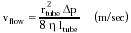{Eqn. 9.26}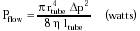{Eqn. 9.27}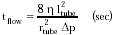{Eqn. 9.28}

Taking h = 0.6915 x 10-3 kg/m-sec for pure water at T = 310 K, a nanotube with rtube = 10 nm, ltube = 1 micron, and Dp = 1 atm passes fluid at 'VHP = 0.6 micron3/sec, vflow = 2 mm/sec, with tflow = 0.5 millisec. The incompressibility assumption generally holds in liquids and in gas flows where Dp << ptube, where ptube is the head pressure at the tube entrance.

The above equations describe the resistance to Poiseuille (laminar) flow in a pipe, which is the minimum of resistance of all possible flows in a pipe.361 If the flow becomes turbulent, the resistance increases. The determinative parameter is a dimensionless quantity called the Reynolds number,1187 NR, which is the ratio of the inertial pressure (~ r vflow2) to the viscous pressure (~ h vflow / rtube) in the flow of a fluid of density r, or: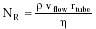{Eqn. 9.29}

For the example of the 10-nm nanotube in the previous paragraph, NR ~ 10-5. A large Reynolds number (Section 9.4.2.1) implies a preponderant inertial effect and the onset of turbulence; a small Reynolds number implies a predominant shear effect (e.g., viscosity) and the maintenance of laminar flow. Reynolds1187 found that the transition from laminar to turbulent flow typically occurred at NR ~ 2000-13,000, depending upon the smoothness of the entry conditions. The lowest value obtainable experimentally on a rough entrance appeared to be NR ~ 2000. However, when extreme care was taken to establish smooth entry conditions (as is more likely in precisely structured nanotubes constructed using molecular manufacturing techniques) the transition could be delayed to Reynolds numbers as high as 40,000.

Even assuming the more conservative NR ~ 2000 figure, it is clear that subsonic flow in nanoscale pipes will almost always be laminar. For a pipe with rtube = 1 micron conveying water at 310 K (r = 993.4 kg/m3), the onset of turbulent flow (taking NR ~ 2000) occurs at vturb (= vflow in Eqn. 9.29) > 1400 m/sec, very nearly the speed of sound in water. In gases or liquids of lower density, or in pipes of narrower bore, or if a less conservative transitional Reynolds number is available due to superior design, then vturb grows still larger. Thus turbulence is primarily a high-velocity, large-tube phenomenon.

In such turbulent flow situations with NR >~ 1000, a well-known empirical formula for the volume flow rate is:363{Eqn. 9.30}

where the turbulence factor Z = 0.005 NR3/4. Thus for NR = 3000, 'Vturb ~ 0.5 'VHP. Nevertheless, even in turbulent conditions of the general flow, fluid motion nearest the tube wall remains laminar in a thin layer of thickness xlam, often estimated as: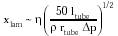{Eqn. 9.31}

For flowing water at 310 K and rtube = 10 micron, ltube = 100 micron, and Dp = 10 atm giving vflow ~ 200 m/sec and NR ~ 3000, then xlam ~ 0.5 microns.

Poiseuille's Law assumes a rigid pipe, an assumption that may not hold for some nanotube designs and which certainly does not hold for human blood vessels, especially the veins. A complete treatment of fluid flow in elastic tubes is beyond the scope of this book. However, for steady laminar flow in an elastic tube with wall thickness hwall, radius rtube, length ltube, p0 and p1 the pressures at the entry and exit ends of the tube, and Ewall = Young's modulus of a Hookean (e.g., a linear force-distance relationship) wall material, then the volume flow rate through the tube may be approximated by:361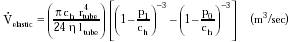{Eqn. 9.32}

where ch = E hwall / rtube. Taking h = 0.6915 x 10-3 kg/m-sec for water at 310 K , hwall = 2 nm, rtube = 10 nm, ltube = 1 micron, p1 = (0.5 p0) = 1 atm, and E = 107 N/m2 (typical for human skin; Table 9.3), then 'Velastic = 0.3 micron3/sec. Care must be taken in applying this formula because many biological tube materials such as blood vessel walls are non-Hookean and exhibit a linear pressure-radius relationship instead. For small elastic deformations, the volume flow rate of such non-Hookean tubes may be approximated by:361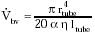{Eqn. 9.33}

where a is the compliance constant, measured experimentally in feline pulmonary veins as 1.98-2.79 m2/N for rtube = 50-100 microns down to 0.57-0.79 m2/N for rtube = 400-600 microns.1190 Other geometric nonuniformities such as regular and irregular tube tapers, embedded resonance chambers, tube bifurcations or multitube confluences have important effects on flow rate but are beyond the scope of this book. Poiseuille's Law also does not strictly hold for fluids seeded with polymers in concentrations as low as 10-4-10-5 by weight, wherein drag may be reduced by a factor of 23.2952,2953

It bears repeating here that as pipes get very small, they can clog more easily due to van der Waals adhesive forces,1152 so it becomes increasingly important to engineer interior vessel surfaces for minimum adhesivity with respect to all materials likely to be transported through the pipes (Section 9.2.3). Bursting strength of fluid-filled pressure vessels is addressed in Section 10.3.1.

Last updated on 20 February 2003